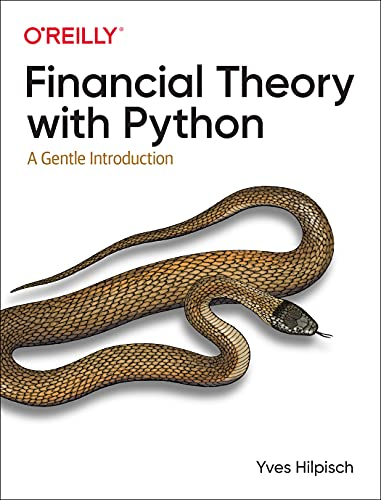# Financial Theory with Python: A Gentle Introduction

• Length: 204 pages
• Edition: 1
• Publisher:
• Publication Date: 2021-10-19
• ISBN-10: 1098104358
• ISBN-13: 9781098104351
Description

Nowadays, finance, mathematics, and programming are intrinsically linked. This book provides the relevant foundations of each discipline to give you the major tools you need to get started in the world of computational finance.

Using an approach where mathematical concepts provide the common background against which financial ideas and programming techniques are learned, this practical guide teaches you the basics of financial economics. Written by the best-selling author of Python for Finance, Yves Hilpisch, Financial Theory with Python explains financial, mathematical, and Python programming concepts in an integrative manner so that the interdisciplinary concepts reinforce each other.

• Draw upon mathematics to learn the foundations of financial theory and Python programming
• Learn about financial theory, financial data modeling, and the use of Python for computational finance
• Leverage simple economic models to better understand basic notions of finance and Python programming concepts
• Use both static and dynamic financial modeling to address fundamental problems in finance, such as pricing, decision-making, equilibrium, and asset allocation
• Learn the basics of Python packages useful for financial modeling, such as NumPy, pandas, Matplotlib, and SymPy